# Planimetrics - math word problems

Study plane measurements, including angles, distances, and areas. In other words - measurement and calculation of shapes in the plane. Perimeter and area of plane shapes.

#### Number of problems found: 2136

• Shooter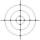The probability that a good shooter hits the center of the target circle I is 0.1. The probability that the target hit intercircle is II 0.58. What is the probability that it hits the target circle I or II?
• Right trianglesHow many right triangles we can construct from line segments 3,4,5,6,8,10,12,13,15,17 cm long? (Do not forget to the triangle inequality).
• Garden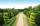The garden has a rectangular shape with lengths of 25 and 40 meters. It has been expanded so that each of its size increased by one fifth. How many square meters increased its acreage?
• Inscribed circle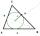Calculate the magnitude of the BAC angle in the triangle ABC if you know that it is 3 times less than the angle BOC, where O is the center of the circle inscribed in the triangle ABC.
• TV towerCalculate the height of the television tower if an observer standing 430 m from the base of the tower sees the peak at an altitude angle of 23°?
• TerezaThe cube has an area of base 256 mm2. Calculate the edge length, volume, and area of its surface.
• Triangular prismCalculate the surface of a regular triangular prism, the edges of the base are 6 cm long and the height of the prism is 15 cm.
• Rectangle - desc circle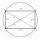Length of the sides of the rectangle are at a ratio 1: 3 . Radius of the circle circumscribed to rectangle is 10 cm. Calculate the rectangle's perimeter.
• Depth angleFrom a cliff of 150 meters high, we can see the ship at a depth angle of 9° at sea. How far is the ship from the cliff?
• Perimeter of RTFind the circumference of the rectangular triangle if the sum of its legs is 22.5 cm and its area is 62.5 cm2.
• Diamond and diagonalsA diamond has diagonals f = 8 cm and g = 6 cm long. How long is this diamond perimeter? (Calculate it!)
• Spherical section cutFind the volume of a spherical section if the radius of its base is 10 cm and the magnitude of the central angle ω = 120 degrees.
• Concrete pedestalThe carpenters made wooden mold on a concrete pedestal in the shape of a cube with an edge 2 meters long. What is the area in which the concrete touches wooden molds? (No lid or bottom)
• Body diagonalThe cuboid has a volume of 32 cm3. Its side surface area is double as one of the square bases. What is the length of the body diagonal?
• Draw triangle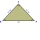Construct an isosceles triangle ABC, if AB = 7cm, the size of the angle ABC is 47°, arms | AC | = | BC |. Measure the size of the BC side in mm.
• TrapezoidHow long are the trapezoid bases with area 24 cm2 and height 3 cm. One base is 3 times longer than the shorter.
• RectanglesCalculate how many squares/rectangles of size 4×3 cm can be cut from a sheet of paper of 36 cm × 32 cm?
• The diamondThe diamond has an area S = 120 cm2, the ratio of the length of its diagonals is e: f = 5: 12. Find the lengths of the side and the height of this diamond.
• Hexagonal pyramidFind the volume of a regular hexagonal pyramid, the base edge of which is 12 cm long and the side edge 20 cm.
• An equilateralAn equilateral triangle is inscribed in a square of side 1 unit long so that it has one common vertex with the square. What is the area of the inscribed triangle?

Do you have an interesting mathematical word problem that you can't solve it? Submit a math problem, and we can try to solve it.

We will send a solution to your e-mail address. Solved examples are also published here. Please enter the e-mail correctly and check whether you don't have a full mailbox.

Please do not submit problems from current active competitions such as Mathematical Olympiad, correspondence seminars etc...

See also more information on Wikipedia.